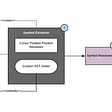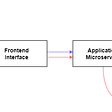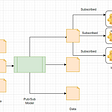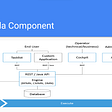# Background

Evaluating the performance of a database usually involves either industry standard testing or or modeling testing based on the business model.

For example, PostgreSQL pgbench supports both tpc-b testing and custom modeling testing; benchmarksql supports tpc-c testing; and gp_tpch supports tpc-h testing.

References :

《TPC-H testing — PostgreSQL 10 vs Deepgreen(Greenplum)》

Testing a sysbench test case with pgbench of PostgreSQL

Analysis of PostgreSQL pgbench SQL RT and transaction RT

Performance evaluation in the database industry_tpc.org

These testing methods can only be used after the database has been built. Is there any way to evaluate performance before building the database?

# What Are the Hardware Indicators That Have the Most Influence on the Performance of a Database?

These indicators have the biggest influence on the performance of a database:

• CPU clock speed
• CPU instruction set
• Number of CPU cores
• Memory clock speed, bus bandwidth
• Hard drive capacity — random IOPS performance
• Hard drive capacity — sequential IOPS performance
• Hard drive bandwidth
• Network bandwidth

For a Greenplum database, the main influencers are:

1.CPU clock speed

Determines the calculation speed of the database. But what operations involve calculations? For example, WHERE clause filtering, operator calculations in a SELECT sub-statement, aggregate calculations, sorting, etc.

2.CPU instruction set

The instruction set determines the performance of certain optimizations in the database. For example, vector computation.

Accelerating OLAP by 10 times through PostgreSQL vectorized execution extension (tiled implementation)

3.Number of CPU cores

CPU clock speed determines the computing capacity of a single CPU core, while the number of CPU cores determines the parallel computing capacity of the database.

4.Memory clock speed, bus bandwidth

When reading/writing in the memory, the memory clock speed and bus bandwidth determine the total read/write capacity, which is a very important factor.

For example, for DDR 2 667, the bandwidth is 64bit×667MHz÷8≈5.3GB/s. If it is a dual channel memory, we have to multiply by 2, so the memory data bandwidth of a dual channel DDR 2 667 is 10.6GB/s.

https://www.cyberciti.biz/faq/check-ram-speed-linux/

https://en.wikipedia.org/wiki/Memory_bandwidth

For example, the maximum memory read/write bandwidth is

`64*2*2400/8/1024= 37.5 GB/sdmidecode --type 17            Array Handle: 0x0034          Error Information Handle: Not Provided          Total Width: 72 bits  ## 带ECC, 64+8          Data Width: 72 bits          Size: 32 GB          Form Factor: DIMM          Set: None          Locator: CPU0_A0          Bank Locator: NODE 1          Type: DDR4          Type Detail:           Speed: 2400 MHz          Manufacturer:           Serial Number:           Asset Tag:           Part Number:           Rank: 2          Configured Clock Speed: 2133 MHz`

Note: This is the technical hard cap of the memory and will not typically be reached by a single CPU core.

What is the computing speed of a single CPU core? We can find out with a simple test.

`Memory speed    #dd if=/dev/zero of=/dev/null bs=4k count=1024000000  ^C68517474+0 records in  68517473+0 records out  280647569408 bytes (281 GB) copied, 34.1855 s, 8.2 GB/s    Block device speed  #dd if=/dev/Block device name of=/dev/null bs=4k count=102300000  ^C2687957+0 records in  2687956+0 records out  11009867776 bytes (11 GB) copied, 4.6525 s, 2.4 GB/s`

In reality, the speed of a single core can’t possibly reach 8.2 GB/s when used in calculations for a database.

5.Hard drive capacity — random IOPS performance

Random IOPS performance will be involved in index access, and (concurrent) access to data in the same hard drive by multiple sessions or processes.

(Cached read can improve the performance of parallel access, nearing sequential IOPS performance.)

6.Hard drive capacity — sequential IOPS performance

Leaving alone concurrencies, an AP system generally reads/writes files in a sequential manner as long as it is not an index scan.

7.Hard drive bandwidth and interface speed

The bandwidth interface speeds of a hard drive determine its maximum data scanning speed.

http://www.shannon-sys.com/product_detail?id=4929256206666909936

Note: this is the technical limit of the hard drive. The computing capacity of a single CPU core cannot reach this limit in most of the cases.

8.Network bandwidth

Network bandwidth determines the data import speed, as well as the redistribution speed during JOIN operations.

A single host may have multiple NICs and data nodes. The network bandwidth is estimated based on the total output bandwidth. For example, if there are ten hosts in a GP cluster and each has two 10 GB NICs, then the total network bandwidth would be 200 GB.

9.Storage skew

The shortcoming of a distributed system is that the slowest node determines the overall processing time. This becomes a larger issue in case of data skew.

The above are the main influencing factors for the performance of a database. Then how can we evaluate the SQL response speed based on these factors?

The cost model of PostgreSQL includes some of these factors, so we can work out the final SQL running cost based on the cost calculation formula and statistical data. If we align the cost with the time, we will be able to see the SQL running time.

Verification of optimizer cost factors — aligning cost constants to timestamps in PostgreSQL

Verification of optimizer cost factors (disk, SSD, memory IO cost calculation) — PostgreSQL real seq_page_cost & random_page_cost in disks, SSD, memory

The above evaluation still requires a database, and data (or statistical information of data) to be imported into the database. How can we evaluate the SQL response time if we only have hardware and data indicators, instead of an actual database? We can take samples of the formula, and then use the database cluster and data indicators as well as our intended SQL requests to evaluate expected performance.

# Example of Greenplum performance evaluation

We can simplify the evaluation model, as the CPU has significant effect (e.g. LLVM, vector optimization, or other optimizations) on the results. Here, I choose to ignore the deviation introduced by the CPU. We will not take into account data skew either.

## 1. Environment

We will discuss how to conduct a performance evaluation with the following environment as an example.

• Hard drive — 2 hard drives, with a read/write bandwidth of 2 GB/s each, to be made into one hard drive through LVM. The total bandwidth is 4 GB/s
• Memory — 512 GB, with a read/write bandwidth of 37.5 GB/s
• CPU — 2.5 GHz, 32-Core
• NIC — Two 10 GB NICs
• Number of machines — 8
• Number of data nodes on each machine — 16 data nodes

## 2. Performance indicators

Performance indicators obtained in another environment

Let’s take integer data for example:

GP column-store

`postgres=# create table mmtest(id int) with (appendonly=true, blocksize=2097152, ORIENTATION=COLUMN);  NOTICE:  Table doesn't have 'DISTRIBUTED BY' clause -- Using column named 'id' as the Greenplum Database data distribution key for this table.  HINT:  The 'DISTRIBUTED BY' clause determines the distribution of data. Make sure column(s) chosen are the optimal data distribution key to minimize skew.  CREATE TABLE    postgres=# insert into mmtest select generate_series(1,100000);  INSERT 0 100000  insert into mmtest select * from mmtest ;  ...  postgres=# insert into mmtest select * from mmtest ;  INSERT 0 409600000    postgres=# select pg_size_pretty(pg_total_relation_size('mmtest'));   pg_size_pretty   ----------------   3133 MB  (1 row)    postgres=# select count(*) from mmtest ;     count     -----------   819200000  (1 row)    Time: 779.444 ms    postgres=# select * from mmtest where id=0;   id   ----  (0 rows)    Time: 422.538 ms`

GP row-store

`postgres=# create table mmtest1(id int)  postgres-# ;  NOTICE:  Table doesn't have 'DISTRIBUTED BY' clause -- Using column named 'id' as the Greenplum Database data distribution key for this table.  HINT:  The 'DISTRIBUTED BY' clause determines the distribution of data. Make sure column(s) chosen are the optimal data distribution key to minimize skew.  CREATE TABLE  Time: 273.659 ms  postgres=# insert into mmtest1 select * from mmtest;    postgres=# select pg_size_pretty(pg_total_relation_size('mmtest1'));   pg_size_pretty   ----------------   28 GB  (1 row)    postgres=# select count(*) from mmtest1 ;     count     -----------   819200000  (1 row)    Time: 1171.229 ms    postgres=# select * from mmtest1 where id=0;   id   ----  (0 rows)  Time: 452.582 ms`

PG row-store

`create unlogged table mmtest(id int);  postgres=# insert into mmtest select generate_series(1,100000);  INSERT 0 100000  insert into mmtest select * from mmtest ;  ...  postgres=# insert into mmtest select * from mmtest ;  INSERT 0 409600000    postgres=# select pg_size_pretty(pg_total_relation_size('mmtest'));   pg_size_pretty   ----------------   28 GB  (1 row)    postgres=# select * from mmtest where id=0;   id   ----  (0 rows)    Time: 56410.222 ms (00:56.410)    32 Parallel computing  3.02 seconds`

## 3. Query performance evaluation

a. Data scanning time

i) Non-memory cache hit:

Scanning speed of each process depends on

• The size of the rows

• Single core processing speed: 40 million rows/s

• Reading speed of a single process: 2.4GB/s.

The longest measured time is used. Max scanning speed of each host is 4GB/s.

least (number of records/(total number of data nodes x 40 million), number of records/(total number of CPU cores * 40 million), table size/(number of data node hosts x 4 GB), table size/(total number of data nodes x 2.4 GB))

ii) Memory cache hit:

Scanning speed of each process depends on

• The size of the rows

• Single core processing speed: 40 million rows/s,

• Reading speed of a single process: 8.2GB/s.

The longest measured time is used. Max scanning speed of each host is 37.5 GB/s. We can calculate the scanning capacity of a single host and the entire cluster based on the number of nodes on each host.

least (number of records/(total number of data nodes x 40 million), number of records/(total number of CPU cores * 40 million), table size/(number of data node hosts x 37.5 GB), table size/(total number of data nodes x 8.2 GB))

iii) OSS scanning capacity

Alibaba Cloud also provides an OSS external table.

The current access speed of a single process on a data node is approximately 30 MB/s. If you open multiple sessions to access OSS at the same time, the speed will see a linear increase.

Therefore, the maximum speed is determined by the NIC bandwidth.

least (number of hosts x NIC bandwidth, number of data nodes x 30 MB/s)

b. Data computing time

Taking integer data for example, the processing speed of a single core is 40 million rows/s.

We can evaluate the processing capacity of the entire HybridDB for PostgreSQL based on the number of data nodes and the processing capacity of a single CPU core.

least (total number of records/(total number of data nodes x 40 million), total number of records/(total number of data node host CPUs x 40 million))

c. Data aggregation time

Taking COUNT aggregation for example, the single core row processing speed is 33 million rows/s.

We can evaluate the processing capacity of the entire HybridDB for PostgreSQL based on the number of data nodes and the processing capacity of a single CPU core.

least (total number of records/(total number of data nodes x 33 million), total number of records/(total number of data node host CPUs x 33 million))

d. Data sorting time

We can evaluate the processing capacity based on the number of data nodes and the processing capacity of a single CPU core.

The time is also affected by work_mem, temporary file write speed, and the sorting method.

e. Data JOIN time

We can evaluate the processing capacity based on the number of data nodes and the processing capacity of a single CPU core.

The time is also affected by the types of JOIN operations, as the methods of evaluating speed for HASH, MERGE, and Nested Loop joins are different.

In a HASH join, each table is scanned once, and the time used for each table is counted.

In a MERGE join, the SORT time for each table is counted.

In a Nested Loop join, the time taken to search each of row in the inner table is counted.

JOIN may also involve data redistribution, in which case the redistribution time needs to be estimated.

f. Data return time

We can evaluate the time based on the network bandwidth of the MASTER node and the return time of a single CPU core.

## 4. Data import performance evaluation

a. Insert a single commit

Concurrent write, less than 10,000 entries/second

b. Insert a single sql statement to batch commit

Concurrent write, less than 100,000 entries/second

c. Insert a transaction statement to batch commit

Concurrent write, less than 100,000 entries/second

d. COPY

Concurrent write, less than 150,000 entries/second

e. OSS

Alibaba Cloud also provides an OSS external table.

The current access speed of a single process on a data node is approximately 30 MB/s. If you open multiple sessions to access OSS at the same time, the speed will see a linear increase.

Therefore, the maximum speed is determined by the NIC bandwidth.

least (number of hosts x NIC bandwidth, number of data nodes x 30 MB/s)

f. gpfdist

Similar to OSS.

## 5.Data redistribution performance evaluation

Data redistribution time evaluation

We can evaluate the data redistribution time based on the total network bandwidth. For example, if network bandwidth for each server is 20 GB, and we have a total of 8 servers, then the total network bandwidth would be 160 GB.

Data redistribution for a table with 16 GB takes 16/(160/8) = 16/20 = 0.8 seconds.

## 6. Data vacuum full (redistribute) performance evaluation

a. vacuum full

Involves data redistribution, so the redistribution time needs to be taken into consideration.

b. alter table redistribute.

If the redistribution key is not changed and data redistribution is not involved, then the operation will be completed within the node.

This is perfect for reducing the size of the expanded data.

# References:

Verification of optimizer cost factors — aligning cost constants to timestamps in PostgreSQL

Verification of optimizer cost factors (disk, SSD, memory IO cost calculation) — PostgreSQL real seq_page_cost & random_page_cost in disks, SSD, memory

Reference:

https://www.alibabacloud.com/blog/Performance-Evaluation-Methods-for-Distributed-MPP-Databases---Best-Practice-for-PostgreSQL_p334288?spm=a2c41.11133674.0.0

--

--

--

## More from Alibaba Cloud

Follow me to keep abreast with the latest technology news, industry insights, and developer trends. Alibaba Cloud website:https://www.alibabacloud.com

Love podcasts or audiobooks? Learn on the go with our new app.

## How to Install OpenLDAP and phpLDAPadmin on Ubuntu 16.04## Xamarin, Sharing is Good## Optimized Bridge Island##  We had a lucky escape## Language Server for Ballerina— Auto Completion Engine in Depth## CS371p Spring 2021: Luca Chaves Rodrigues Noronha dos Santos## build a minimal docker image## Alibaba Cloud

Follow me to keep abreast with the latest technology news, industry insights, and developer trends. Alibaba Cloud website:https://www.alibabacloud.com

## Confluent Kafka Multi-Region Cluster in 2 minutes## A recommended resilient and scalable Elasticsearch data indexing architecture — Part 2## Kafka: A general understanding## Single Source of Truth : Business Process Automation With Camunda